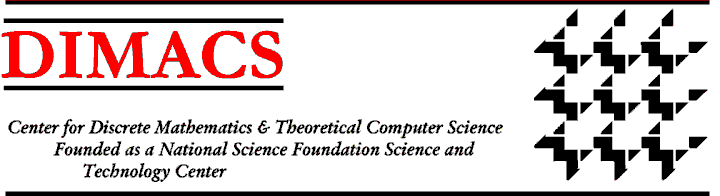### DIMACS Workshop on Probabilistic Analysis of Algorithms

#### May 11-14, 1997 Princeton University

Organizers:
Alan Frieze, Carnegie Mellon, af1p+@andrew.cmu.edu
Michael Molloy, University of Toronto, molloy@cs.toronto.edu
Presented under the auspices of the DIMACS Special Focus on Discrete Probability.

### Theme:

Problems arising in the mathematical analysis of algorithms have provided an important stimulus for the growth in interest in Discrete Mathematics. Probabilistic techniques have an important role in this endeavour. The theory of algorithms has undergone an extraordinarily vigorous development over the last 20 years or so, and probability theory has emerged as one of its most vital partners. By its nature probabilistic analysis cuts across the boundaries of Computer Science, Discrete Mathematics, Operations Research and Probability Theory.

This workshop focusses on the analysis of algorithms in the {\em average case}, assuming a probabilistic distribution on input instances. The goal is to obtain good estimates of various performance measures of algorithms such as solution quality and running time. The main objective is to explain why many simple algorithms, often used in practice, perform much better than as predicted by worst-case analysis. Pathological cases are generally rare and simple algorithms can often be shown to perform well in the average case. This is especially true in the case of NP-complete problems where it seems that the probabilistic approach may be the best way and indeed may be the only way to understand the success of heuristic combinatorial algorithms.

We identify five major sub-areas as the focus of the workshop.

Algorithmic Theory of Random Graphs and Related Combinatorial Problems.
Analysis of Euclidean Functionals.
Linear Programming and Integer Programming Problems.
Analysis of Fundamental Algorithms.
Average Case Completeness

The workshop will bring together leading researchers in the Analysis of Algorithms along with younger researchers in the area. There should be about 15-20 lectures presenting progress, trends and open problems together with ample time for discussion. We believe that bringing together researchers from these different but closely related areas will result in an interesting cross-fertilisation of ideas.Next: Call for ParticipationIndexDIMACS Homepage
Contacting the Center